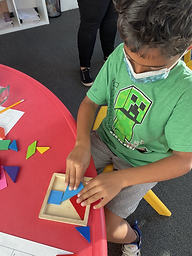## Ms. Caitlyn

### Target 1​

###### Lesson Type:

New

Number Operation

:

Computation

Solve multi-step word problems within 1,000,000 (addition, subtraction, multiplication, and division).

###### 1:

Identify the correct arithmetic processes based on the information presented in word problems.

###### 2:

Find the key words in word problems that indicate the correct arithmetic process to use: Addition: add, altogether, both, combined, in all, plus, sum, total; Subtraction: difference, fewer, how many/much more, left, less, minus, remains; Multiplication: times, product, each, twice; and Division: how many in each, divided by, quotient, goes into, split evenly.

###### 3:

Find and use the needed information in a word problem to solve.

4th

###### Vocabulary:

CUBES Method, Math Action Words, Key Numbers, Relevant Information

Activities:

Students brainstormed "math action words" that tell them what operation to use in a problem. They wrote their ideas on Post-Its and stuck them underneath the corresponding category around the room.

Students used the CUBES method to solve word problems: (C)ircle the numbers, (U)nderline the question, (B)ox the math action words, (E)liminate extra information, and (S)olve and show your work.### Home Exploration

###### Challenge Problem:

Students were sent home with the other half of their worksheet. There are four problems in the back that are yet to be solved.

N/A

###### Guiding Questions:

What are the math action words that tell us what operation to use? (What is happening in the problem?)

What are the key numbers? What do these numbers tell us?

Encourage students to solve problems step-by-step (taking the problem line-by-line, as to not forget important numbers or information).

N/A## Absent Students:

### Target 2

:

###### 1:

Look at a visual puzzle to analyze the pieces and determine which is the correct piece to choose.

###### 2:

Look at a visual-spatial puzzle to analyze the pieces and determine which is the correct piece to choose.

###### 3:

Identify given relationships between figures and select figures that have an identical relationship.

5th

###### Vocabulary:

Spatial Reasoning, Visual Reasoning, Shapes, Space, Composite

Activities:

Students reviewed solving tangram puzzles.

Students filled in abstract silhouettes with shapes and competed through different levels of difficulty.### Home Exploration

###### Guiding Questions:### Target 3

:

###### Vocabulary:

Activities:### Home Exploration